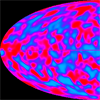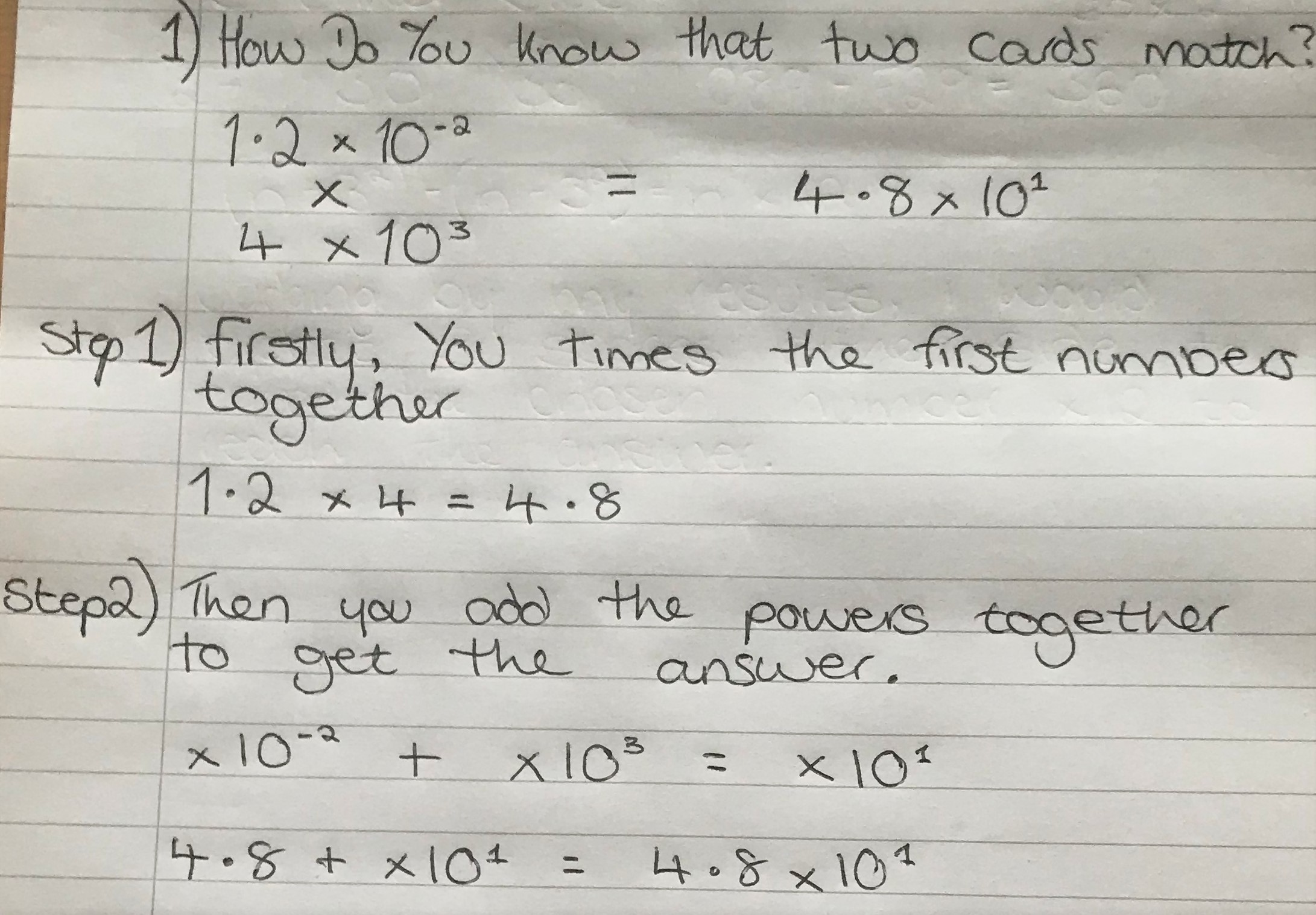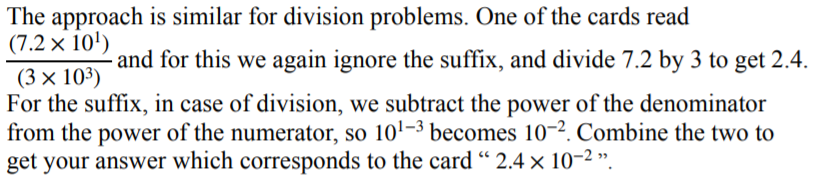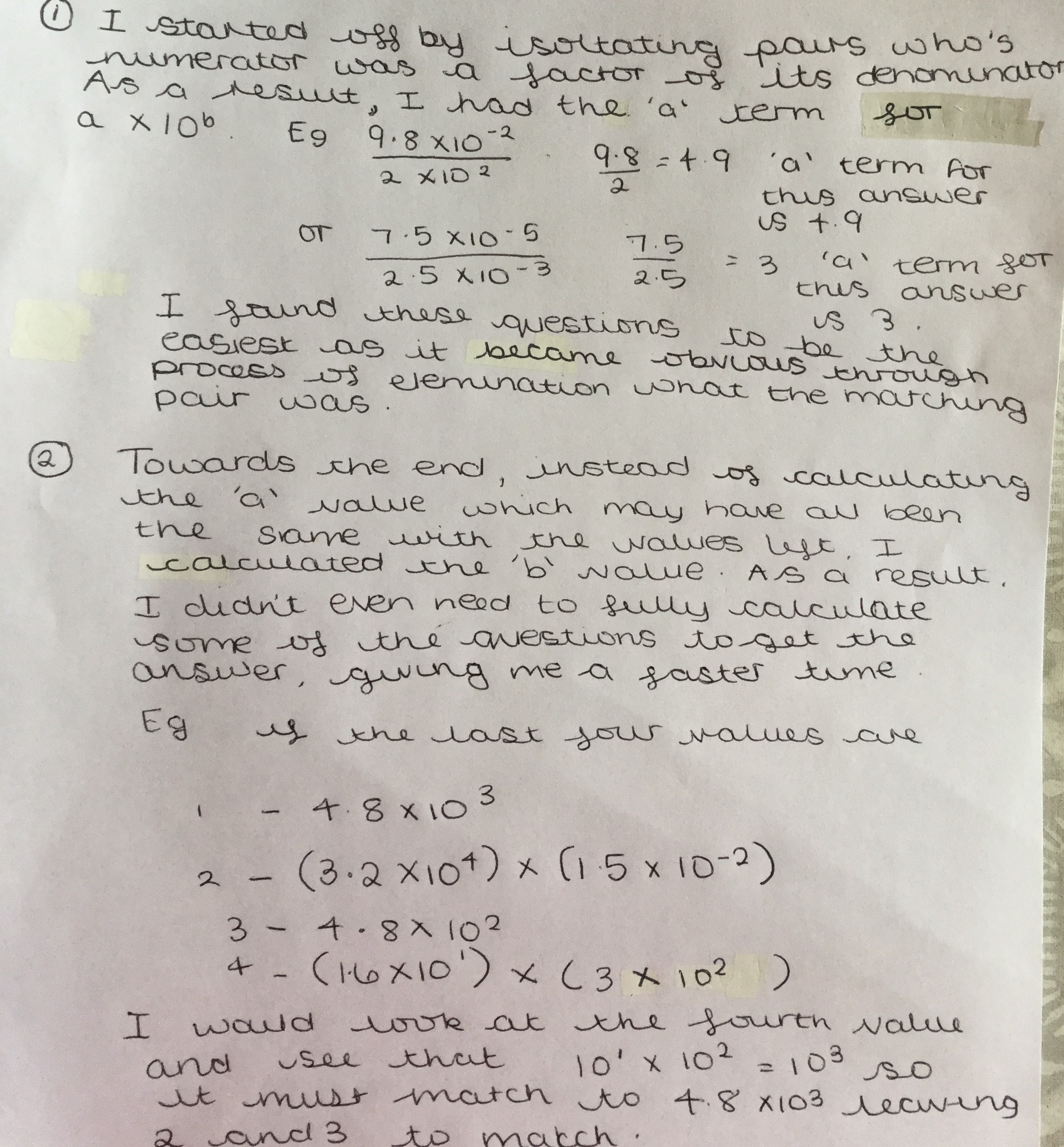#### You may also like### Big and Small Numbers in Physics

Work out the numerical values for these physical quantities.### Big and Small Numbers in the Physical World

Work with numbers big and small to estimate and calculate various quantities in physical contexts.

# Standard Index Form Matching

##### Age 14 to 16Challenge Level

Hugo from Bangkok Patana School in Thailand, Freya and Jonty from Long Field Academy in the UK, Kota from Latymer School in England and Arsh from Jamnabai Narsee International School in India explained how you can tell that two cards match. This is some of Jonty's work:This is some of Arsh's work:Kota wrote all of that algebraically:

When you multiply two standard forms together, the answer will become the product of the coefficients, and the index will be the sum of the two indices.
So $$a\times10^x \hspace{2mm}\times\hspace{2mm} b\times10^y = ab\times10^{x+y}$$
Similarly $$\frac{a\times10^x }{ b\times10^y} = \dfrac{a}{b}\times10^{x-y}$$

Deeya from St Mary's Catholic High School Astley Manchester in England used this strategy to match the cards quickly:Ariel started from the second part of Deeya's strategy:

For level 1, modifying of index is not needed. Therefore you can match some unique index ($b$) first, then multiply the $a$ to identify the rest. Example:
Four of the cards are $\left(2.4\times10^{-5}\right)\times\left(2\times10^4\right),$ $2.4\times10^{-2},$ $\dfrac{7.2\times10^1}{3\times10^3}$ and $4.8\times10^{-1}.$
$-5+4=-1, 1-3=-2,$ so the first should match the fourth and the second should match the third.

For level 2, it is similar. Except you need to divide the $a$ by $10$ if $a$ is larger than $10$ (or less than $1$)
Example (level 2):
Four of the cards are $\frac{1.5\times10^{-4}}{7.5\times10^{-6}},$ $2\times10^1,$ $\frac{4.5\times10^{-3}}{1.5\times10^{-5}}$ and $3\times10^2.$
Both pairs have a original index of $2,$ (since $-4--6=2$ and $-3--5=2$) so comparison of $a$ is needed.
$1.5\div7.5$ is $0.2,$ so the actual index of the first card is $2-1=1$ (because $0.2\times10^2 = 2\times10^1$)
Therefore the first matches the second and the third matches the fourth.

Mahdi from Mahatma Gandhi International School in India described a similar strategy, but startest with the hardest cards instead of the easiest ones:

The key strategy here I used was to boil down the possibilities every time two cards matched. Throughout the games, I observed that half of the card is simplified and half weren't. Thus over time, I started to take the hardest one (not- simplified) and simplify that using the powers of 10. Mainly I did this but also started to find some connection between the numbers in the numerator and the denominator. So now I had simplified one card and approximated it in less than 3-4 seconds. Next, I looked for the simplified cards and tried to match the powers. There were most of the times two cards that had matching powers of 10, so I used the simplified numbers in division or multiplication (numerator and the denominator) to boil down that as well. So now I had a matching pair in less than 6-7 seconds. I continued doing this (starting to find hardest and matching it with one of the simplified ones) and quickly the possibilities of matching 10 powers reduce. Then  I could easily find the matching pairs by just approximating powers of 10. I did not have to be precise with division or multiplication. One key thing I had to remember was that sometimes the numbers in the numerator and denominator also changed the powers of 10, so I had to keep that in mind.